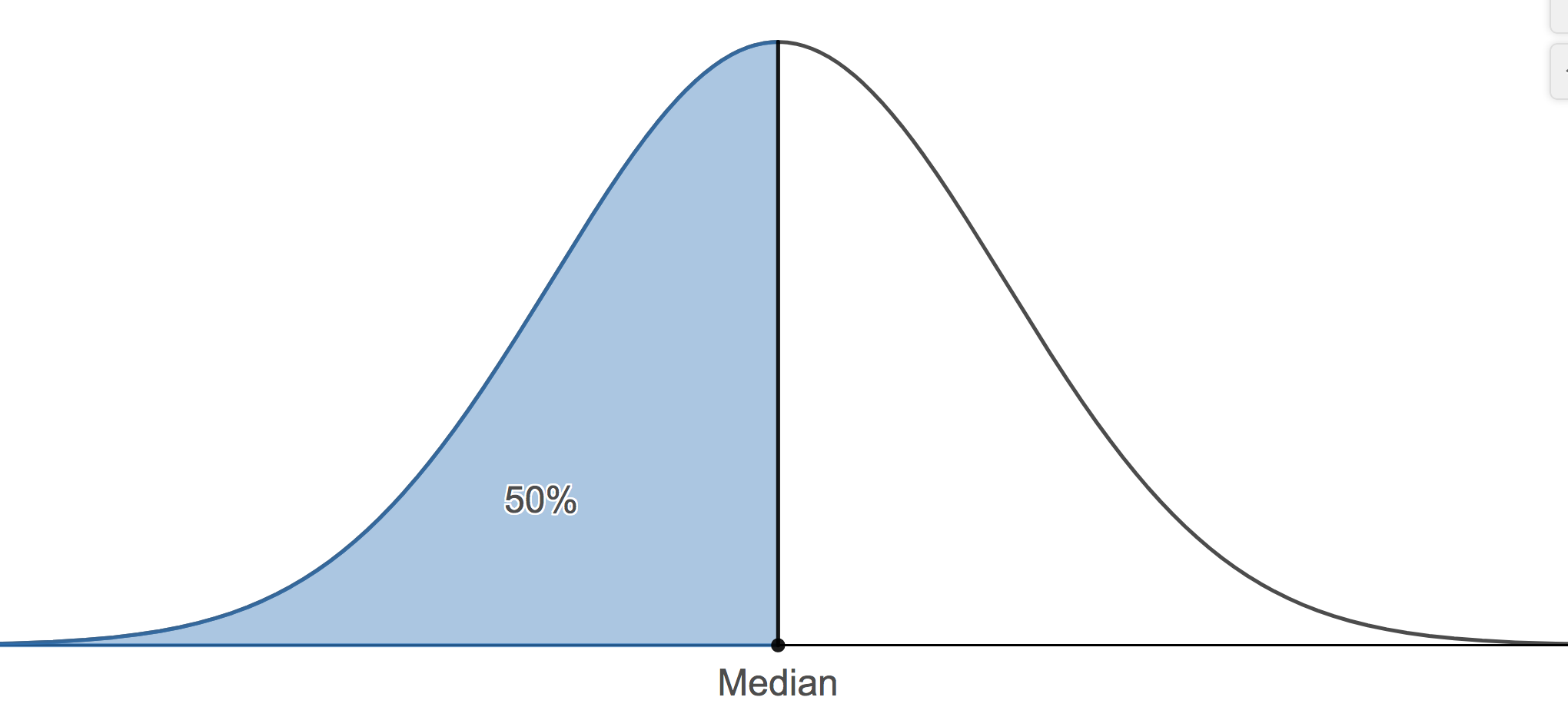# 2.4 - Measures of Position

Measures of position give a range where a certain percentage of the data fall. We briefly referred to percentiles in the last section, but we will also add quartiles to this overview.

Percentiles
The pth percentile of the data set is a measurement such that after the data are ordered from smallest to largest, at most, p% of the data are at or below this value and at most, (100 - p)% at or above it.

A common application of percentiles is their use in determining passing or failure cutoffs for standardized exams such as the GRE. If you have a 95th percentile score then you are at or above 95% of all test takers.

The median is the value where fifty percent or the data values fall at or below it. Therefore, the median is the 50th percentile.We can find any percentile we wish. There are two other important percentiles. The 25th percentile, typically denoted, Q1, and the 75th percentile, typically denoted as Q3. Q1 is commonly called the lower quartile and Q3 is commonly called the upper quartile.## Finding Quartiles Section

The method we will demonstrate for calculating Q1 and Q3 may differ from the method described in our textbook. The results shown here will always be the same as Minitab's result. The method here is also different than from the method presented in many undergraduate statistics courses. This method is what we require students to use. There are two steps to follow: Find the location of the desired quartile If there are n observations, arranged in increasing order, then the first quartile is at position $$\dfrac{n+1}{4}$$, second quartile (i.e. the median) is at position $$\dfrac{2(n+1)}{4}$$, and the third quartile is at position $$\dfrac{3(n+1)}{4}$$. Find the value in that position for the ordered data.

Note! If the value found in part 1 is not a whole number, interpolate the value.